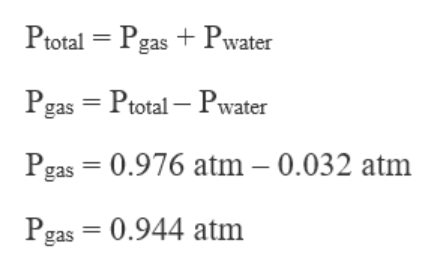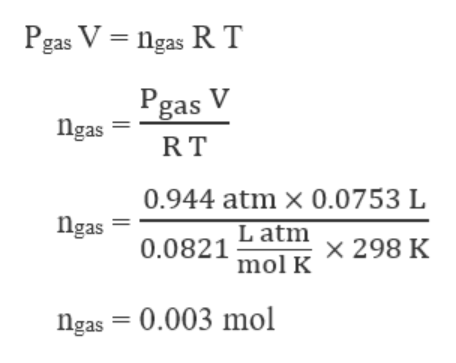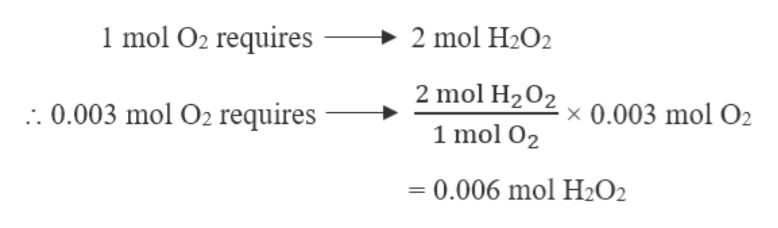# The catalytic decomposition of hydrogen peroxide yields oxygen gas and water, according to the reaction given below. In an experiment, the decomposition of hydrogen peroxide yielded 75.3 mL of gas collected over water at 25°C and 0.976 atm. (Pwater = 0.032 atm at 25°C)2 H2O2(aq) → 2 H2O(l) + O2(g)If the original H2O2 solution had a volume on 500 mL, what was the molar concentration of hydrogen peroxide prior to decomposition?

Question
89 views

The catalytic decomposition of hydrogen peroxide yields oxygen gas and water, according to the reaction given below. In an experiment, the decomposition of hydrogen peroxide yielded 75.3 mL of gas collected over water at 25°C and 0.976 atm. (Pwater = 0.032 atm at 25°C)

2 H2O2(aq) → 2 H2O(l) + O2(g)

If the original H2O2 solution had a volume on 500 mL, what was the molar concentration of hydrogen peroxide prior to decomposition?

check_circle

Step 1

Given,

Volume of gas, O2 collected over water, V = 75.3 mL = 0.0753 L   (1 mL = 0.001 L)

Temperature, T = 25°C = 273 + 25 = 298 K

Total pressure, Ptotal = 0.976 atm

Vapour pressure of water at 25°C, Pwater = 0.032 atm

Original volume of H2O2 solution = 500 mL = 0.5 L

The pressure of gas, O2 can be calculated from Dalton\'s Law of Partial Pressures, which states :help_outlineImage TranscriptionclosePtotal = Pgas +Pwater Pgas = Ptotal – Pwater Pgas = 0.976 atm – 0.032 atm Pgas = 0.944 atm fullscreen
Step 2

The moles of gas, O2 can be calculated using the ideal gas equation as :help_outlineImage TranscriptionclosePgas V = ngas R T Pgas V Ngas RT 0.944 atm × 0.0753 L Ngas L atm x 298 K 0.0821 mol K ngas = 0.003 mol fullscreen
Step 3

The catalytic decomposition of hydrogen peroxide yields ox...help_outlineImage Transcriptionclose1 mol O2 requires → 2 mol H2O2 2 mol H202 1 mol O2 :. 0.003 mol O2 requires x 0.003 mol O2 = 0.006 mol H2O2 fullscreen

### Want to see the full answer?

See Solution

#### Want to see this answer and more?

Solutions are written by subject experts who are available 24/7. Questions are typically answered within 1 hour.*

See Solution
*Response times may vary by subject and question.
Tagged in

### Alcohols, phenols and ether